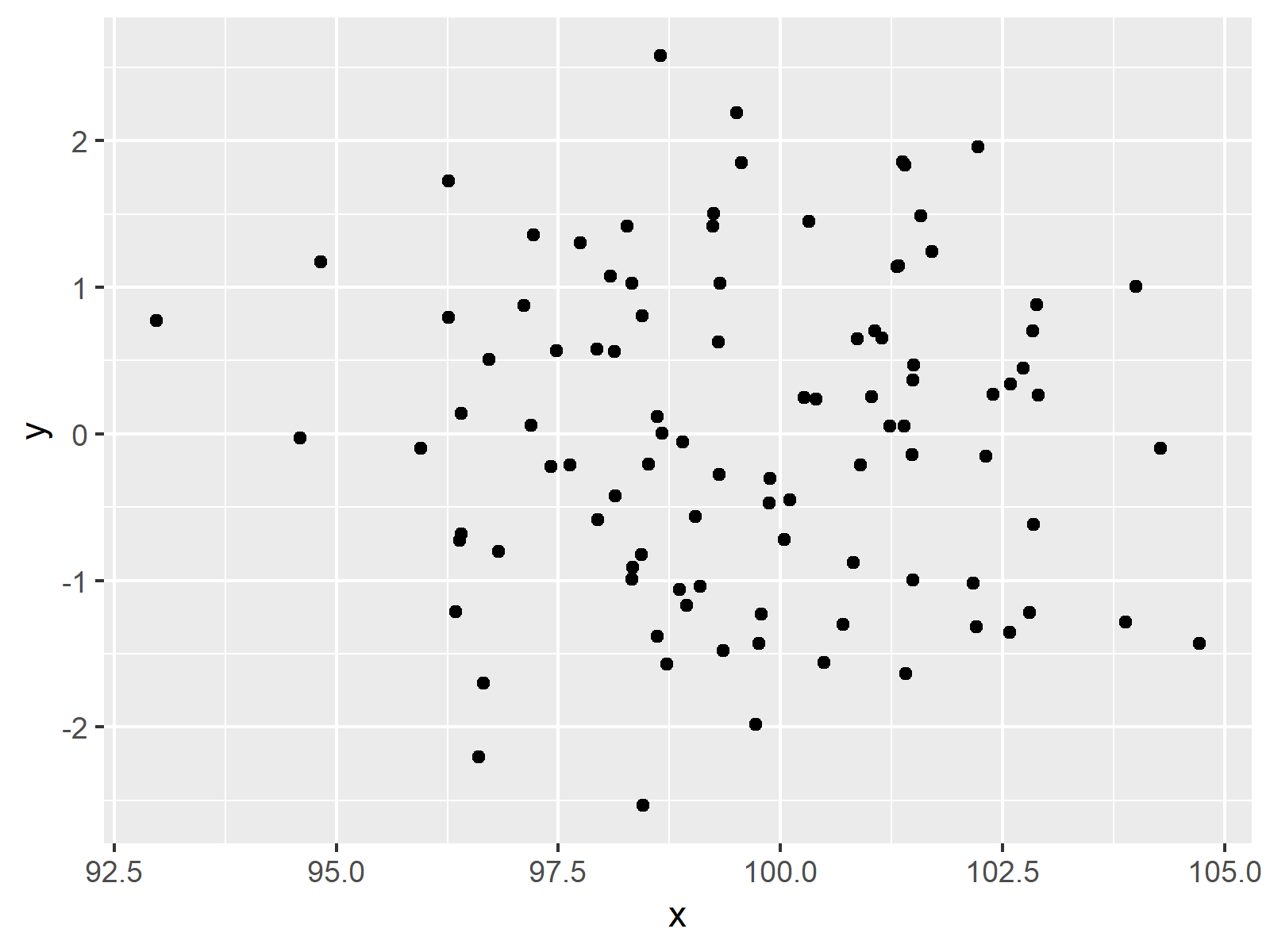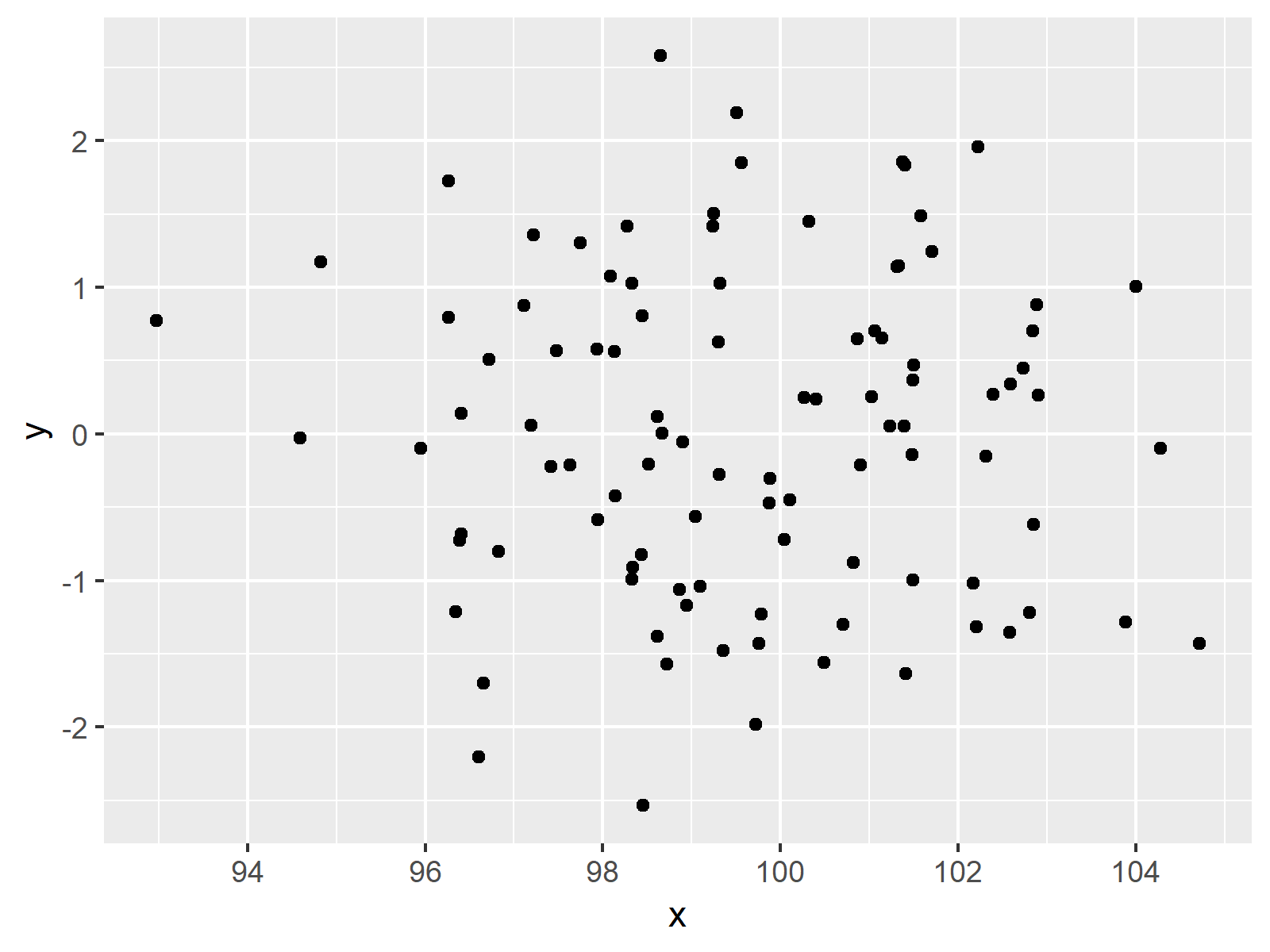# Display Only Integer Values on ggplot2 Axis in R (Example)

In this tutorial you’ll learn how to draw a ggplot2 axis containing only integers in R.

The content of the page is structured as follows:

Let’s dive right in.

## Example Data, Packages & Default Plot

First, we’ll have to create some data that we can use in the examples later on:

```set.seed(10821) # Create data data <- data.frame(x = rnorm(100, 100, 2), y = rnorm(100)) head(data) # Head of data # x y # 1 100.31996 1.45011641 # 2 101.13881 0.65336914 # 3 96.82407 -0.80131958 # 4 100.10791 -0.44847950 # 5 94.59277 -0.02716243 # 6 101.58426 1.48654344```

As you can see based on the previous output of the RStudio console, our example data consists of two columns both containing random numeric values.

If we want to create a plot of our data with the ggplot2 add-on package, we also have to install and load ggplot2:

```install.packages("ggplot2") # Install & load ggplot2 library("ggplot2")```

Next, we can plot our data:

```ggp <- ggplot(data, aes(x, y)) + # Create default ggplot2 graph geom_point() ggp # Print plot```The output of the previous code is shown in Figure 1 – A default ggplot2 plot with non-integer values on the x-axis.

## Example: Display Prettier Axis Values Using scales Package

This Example explains how to remove non-integer numbers from ggplot2 axes in R. First, we have to install and load the scales package:

```install.packages("scales") # Install & load scales library("scales")```

Now, we can use the pretty_breaks function of the scales package to modify our axis values.

```ggp + # Improve scales scale_x_continuous(breaks = pretty_breaks())```As shown in Figure 2, the previous syntax created a ggplot2 scatterplot showing only integer numbers as axis breaks.

## Video & Further Resources

Do you need further explanations on the R programming syntax of this page? Then you could watch the following video of my YouTube channel. I illustrate the content of this article in the video:

Besides that, you may read the other tutorials of my website.

At this point you should know how to create a ggplot2 axis with only integer values in the R programming language. Let me know in the comments, if you have further questions.